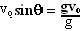## Saturday, November 24, 2007

### Irodov Problem 1.96

a) Force is rate of change of momentum and here the force if constant equal to mg. In other words the particle's momentum changes at a constant rate of mg. Clearly in an interval of t seconds the momentum change will be mgt.

b)The component of velocity of the particle along the direction of gravity will be. Here, g is the vector of gravitational acceleration, g is its magnitude, vo is the velocity vector and vo is its magnitude. It can be found in any standard physics book (in projectile motion) that the total time before the particle hits the ground is given by. As in the part a), the rate of change in momentum is constant and equal to mg.
Thus the total change in momentum is given by.